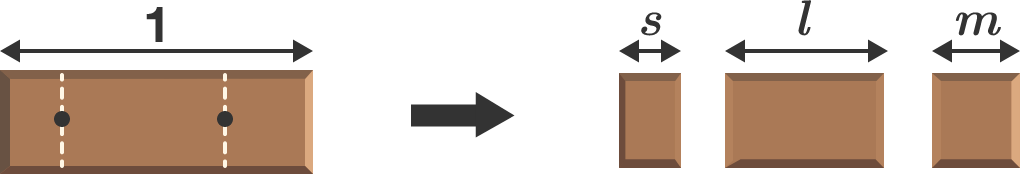# Chocolate Breaker

You have many chocolate bars of unit length and start breaking each of them into 3 pieces by randomly choosing two points on the bar. What are the average lengths of the shortest, medium, and longest pieces?

If the product of these averages can be expressed as $\frac pq$, where $p$ and $q$ are coprime positive integers, give your answer as $p+ q$.×